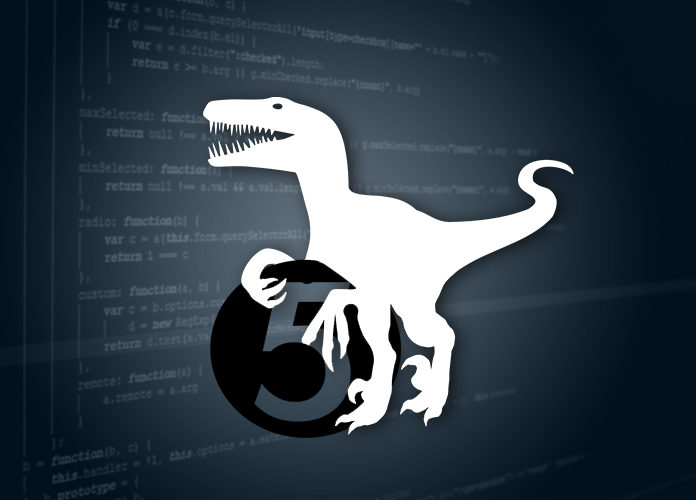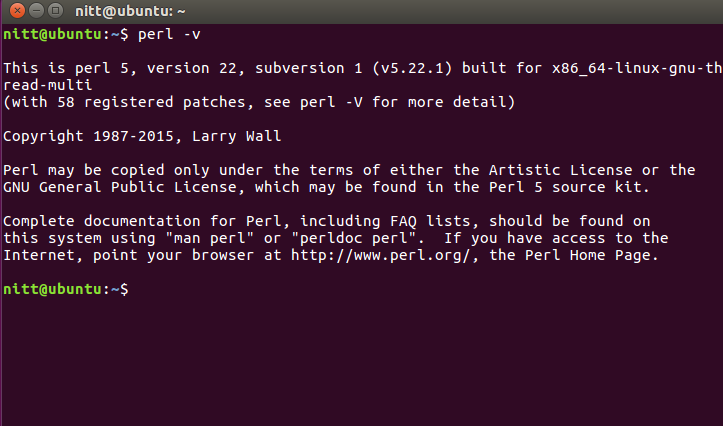# Introduction To Perl 5 And Syntax In Detail With Examples

0
1264Perl is a popular programming language. Its full form is “Practical Extraction and Reporting Language” Larry Wall created Perl in 1987. He created it when working on a bug reporting system and AWK programming language. To his surprise, he didn’t find AWK useful, and that’s why he created Perl in the first place.

## Perl 5 Introduction

PERL is best defined as a high-level, dynamic, interpreted programming language. It is best suited for text editing purposes. PERL soon caught the attention of the programmers worldwide and can be used for multiple projects including network, web app development and so on. Let’s start learning more about Perl and its syntax below.

### Perl 5 Syntax in Detail and with examples

Installing Perl 5 – Linux

We are going to install Perl 5. For tutorial purposes, we are only covering Linux installation. If you are using Windows, you can follow this installation guide. We are using Linux 16.04 LTS version for the tutorial.

For Linux users, you probably have Perl pre-installed. To check if it installed in your Linux, run the following command. Perl also comes pre-installed on Mac OS. If it runs successfully, you will get the following response.Now, we have to update Perl before starting to use it. To do so, run the following command in the command line.

sudo apt-get install perl
Perl First Program: Hello World
Let’s write a hello world program in Perl.

```#!/usr/bin/perl -w
#this is just a comment…
print "Hello World";```

The first line of the code tells to IDE to execute the PERL file so that we run the program. You can also add the line into the interpreter path.

The last line is a print command that prints out “Hello World” to the screen.

Perl Variable

Variables are simple containers which can hold values on their own. The values can be overwritten. In Perl, there are three types of variables. They are scalar, arrays, and hashes.

Scalar: It is a variable type that holds only single value. It starts with a “\$” symbol. For example, \$variablename, \$name, \$address, and so on. Also, there are two types of scalar data types: numbers and strings. Numbers contain simple integer values, whereas strings contain string literals or a group of letters.

Perl Array

An Array is a type of variable that stores data in a list. To access the values, index numbers are used. You can store any type of data in Array including floating values, numbers, strings and so on. It starts with the “@” symbol. For example,

`my @exp_array;`

It defines an Array named “exp_array”

Now, let’s store value to it.

`@exp_array=(a,b,c,d)`

The index starts from 0. This mean if you call \$my_array. It will return “a”.

```Similarly, \$exp_array = b
\$exp_array = c
\$exp_array = d```

You can also created dynamic arrays. Let’s do it below.

`my \$string = “example of dynamic array”;`
```my @array;
@array = split(‘a’,\$string);
foreach(@array)
{
print “\$_ \n”;
}```

Output: ex
Mple of dyn
mic
rr
y

The above code splits the array according to the “a” delimiter.

Perl Hashes
Perl hashes is a key, value-based type data type. This means that it doesn’t use array index. Values are stored corresponding to key values. Let’s look at an example.

print %hash = (“Nitish” => 30, “Singh” => 23, “Monu” = “22”);

Now if we print \$hash{‘Nitish’}, it will return 30.

Perl Conditional Statements

Conditional statements are used to make decisions based on logic. For example, you would buy 5 fruits only if the cost of each fruit is less than \$1. If it is higher than that, you will buy only 4. This type of logic can be used in your programs. Let’s look at one Perl conditional statements below.

Perl If

```my \$x = 1;
if (\$x==1)
{
print “I will buy 5 fruits”;
}```

Output: I will buy 5 fruits

Perl If Else
my \$x = 2;
if (\$x==1)
{
print “I will buy 5 fruits”;
}
else
{
print “I will buy 4 fruits.”
}

Output: I will buy 4 fruits.

Perl Elseif

```my \$x = 2;
if (\$x==1)
{
print “I will buy 5 fruits”;
}
else if(\$x <1.5)
{
print “I will buy 3 fruits.”
}```

Output: I will buy 3 fruits.

Perl Loops
If a logic needs to be repeated, then we use loops. Let’s look at the example below.

```my @array=(1..10);
for(my \$cout=0;\$cout<10;\$cout++)
{
print "\$array[\$cout]";
print "\n";
}```

Output: 1
2
3
4
5
6
7
8
9
10
ou can also foreach to access each elements one by one.

```my @marray=(1..10);
foreach my \$value (@marray)
{
print "\$value\n";
}```

Output: 1
2
3
4
5
6
7
8
9
10

Perl Operator

An operator is an action that is performed on values or variables. It is similar to other programming languages. For now, Perl supports arithmetic, assignment, logical and relational operators.

Arithmetic: +,-,*, /, **, %, ++, —

Example:

```my \$a=10;
my \$b=2;
my \$c;
\$c=\$a+\$b;
\$c=\$a-\$b;
print ("Sub: \$c \n");
\$c=\$a*\$b;
print ("Mul: \$c \n");
\$c=\$a/\$b;
print ("Div: \$c \n");
\$c=\$a**\$b;
print ("Exp: \$c \n");
\$c=\$a%\$b;
print ("Mod: \$c \n");

Output:
12
8
20
5
100
0```

Assignment: +=, -=, *=, /=, **=, %=

Example:

my \$a=10;
\$a+=5;
\$a-=5;
print(“Sub: \$a\n”);
\$a*=5;
print(“Mul: \$a\n”);
\$a/=5;
print(“Div: \$a\n”);

Output

Sub = 10
Mul = 50
Div = 10

Relational and logical: == or eq, != or ne, > or gt, =or ge, || or or, &&or and

my \$a=4;
my \$b=4;
if(\$a == \$b){
print (“True \n”);
}
else{
print (“False \n”);
}
\$a=5;
\$b=6;
if(\$a != \$b){
print (“True \n”);
}
else{
print (“False \n”);
}
if(\$b > \$a){
print (“True\n”);
}
else{
print (“False \n”);
}
if(\$a < \$b){ print ("True \n"); } else{ print ("False \n"); } if(\$a <= \$b){ print ("True \n"); } else{ print ("False \n"); } if(\$b >= \$a){
print (“True \n”);
}
else{
print (“False \n”);
}

Output
True
True
True
True
True
True
True

Conclusion

We hope that you liked a simple introduction and a detailed explanation of some of the key syntax related to Perl 5. So, are you picking up Perl 5 for your next project? Comment below and let us know.